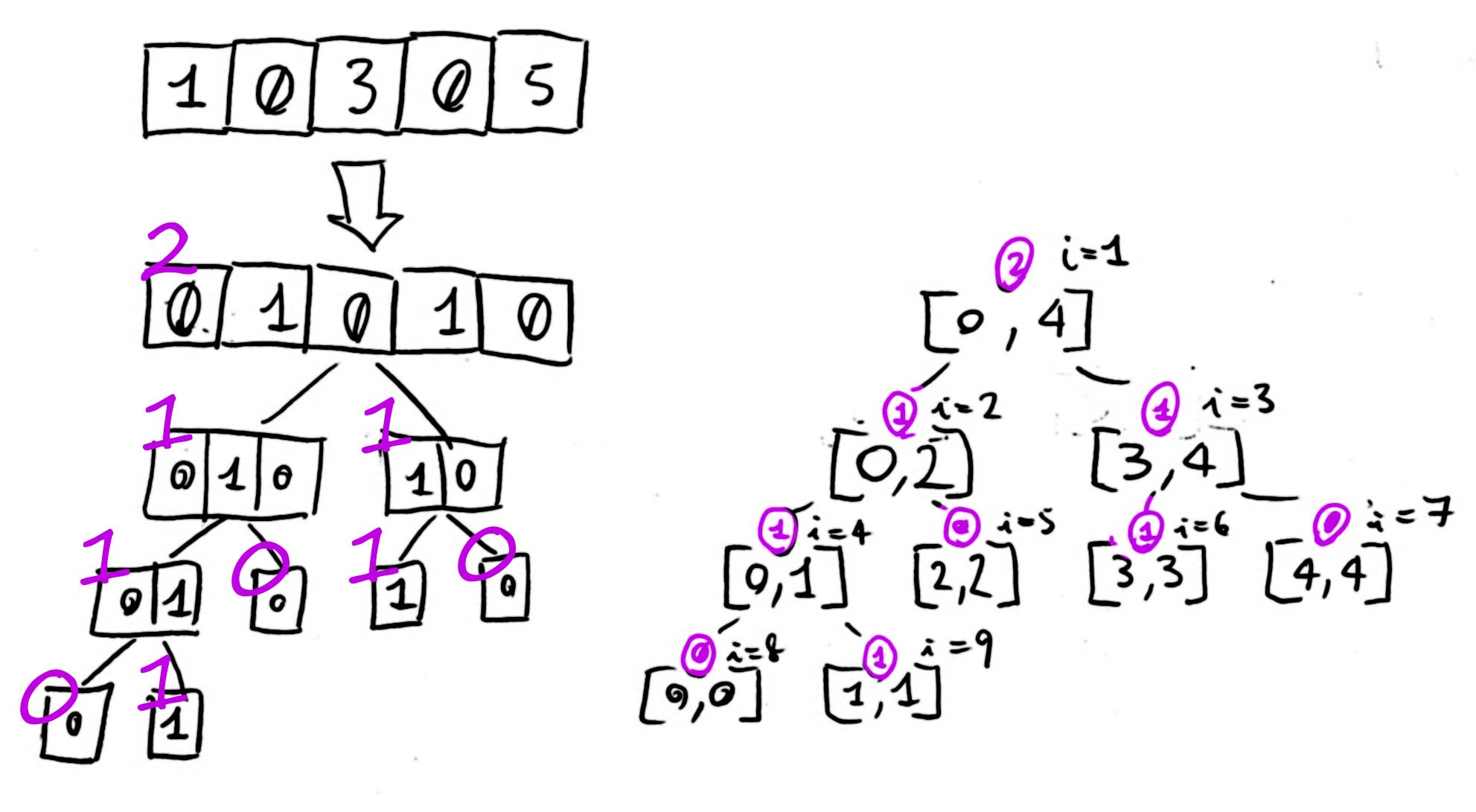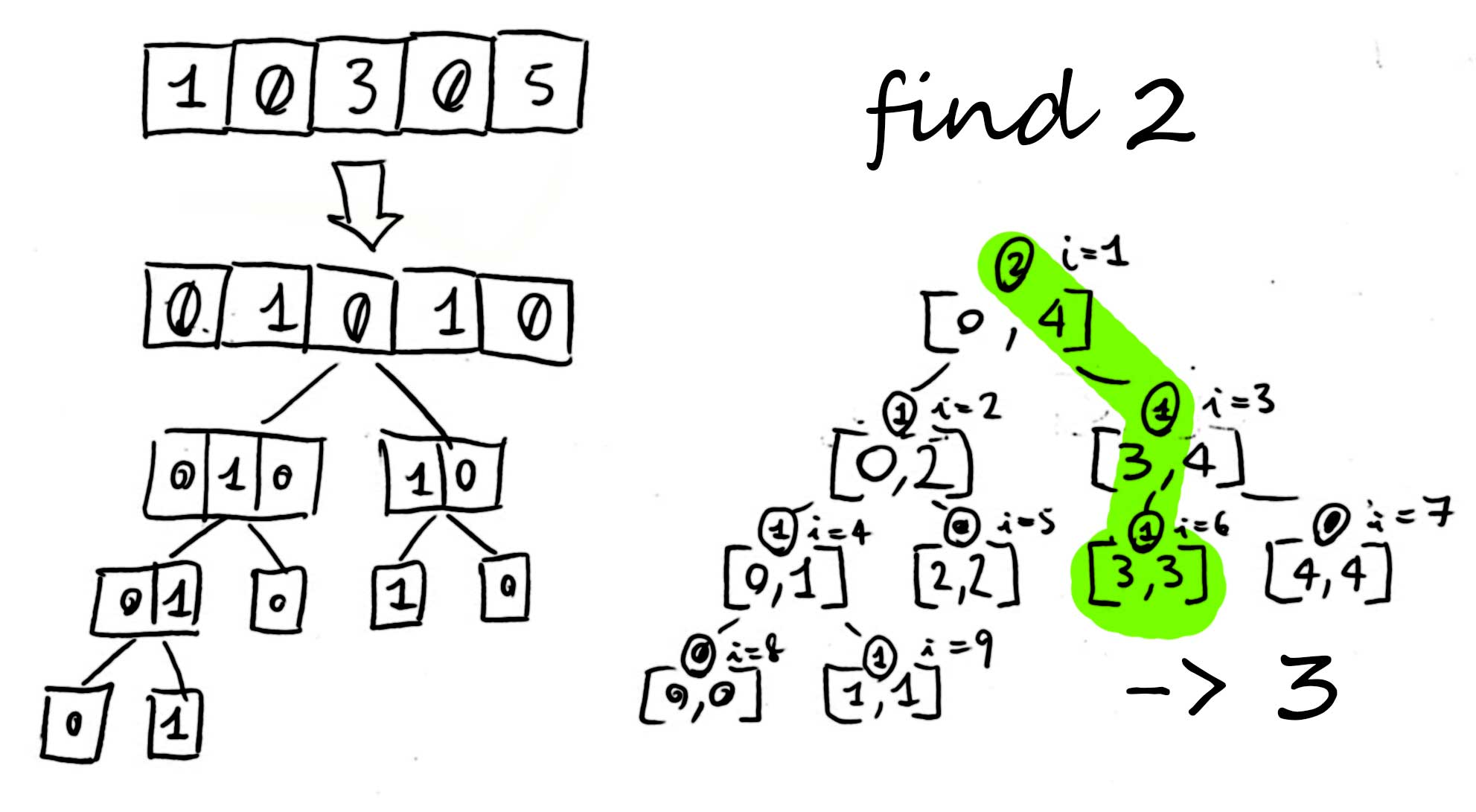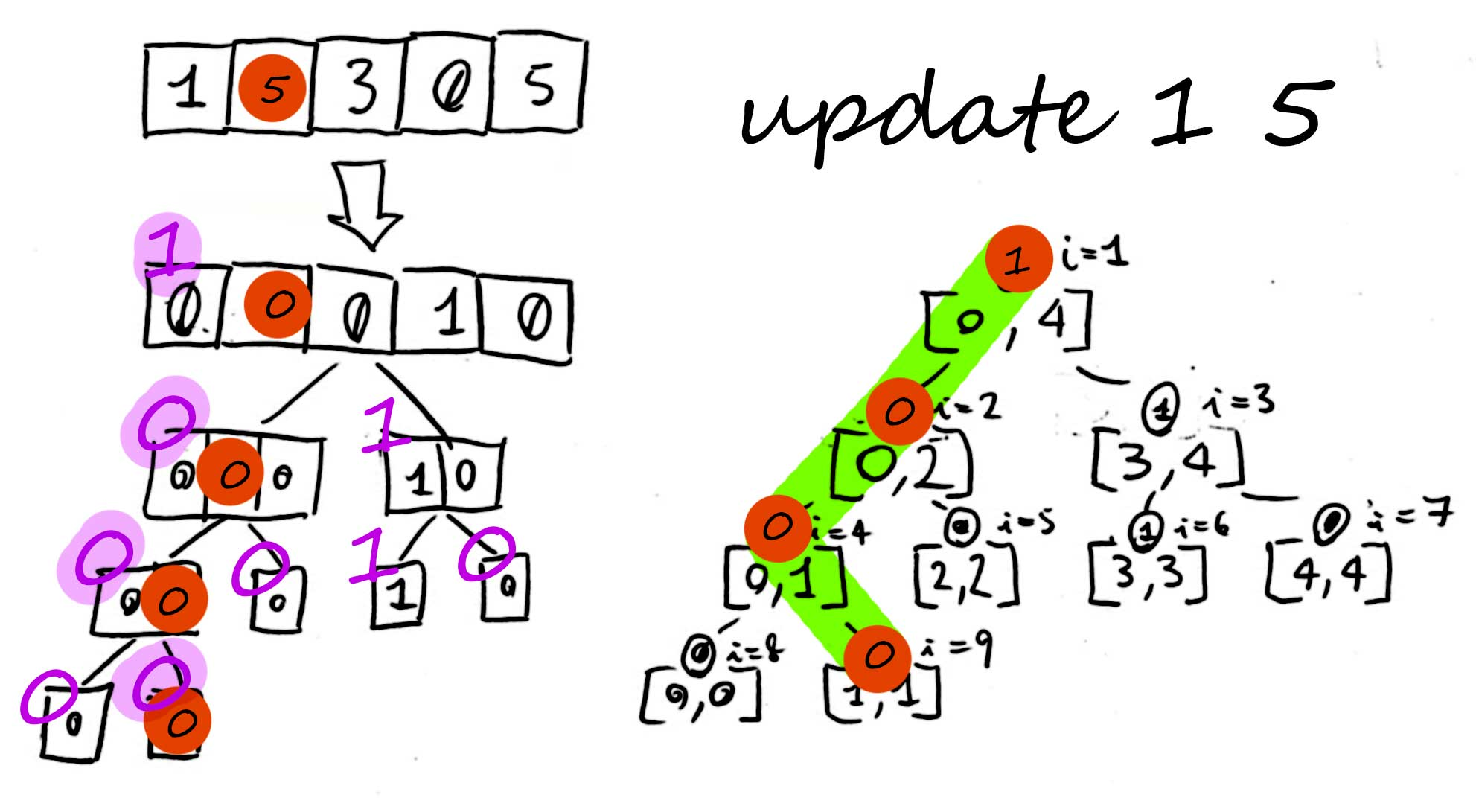# Find Kth Zero

See the original problem on HackerRank.

Consider an array of integers A = [a1, a2, …, aN]. We can perform two types of queries on A:

• 1 k: For query type 1, find and print an integer denoting the index of the kth zero in array A on a new line; if no such index exists (i.e., there is no zero), print NO instead.

• p x: For query type 2, replace the element positioned at index p with integer x (i.e., set A[p] = x).

Given A’s initial state and queries, perform each query in order. For each query of type 1, print the query’s answer on a new line.

### Input Format

The first line contains two space-separated integers describing the respective values of n (the size of the array) and m (the number of queries). he second line contains space-separated integers describing the respective elements of array A (e.g. a0, a1, …, aN).

Each of the m subsequent lines describes a query in one of the following forms:

• 1 k, where 1 is the query type and k denotes the kth zero you must locate an index for in the array.

• 2 p x, where 2 is the query type, p is the index (position) you must update, and x denotes the new value to store at A[p].

### Constraints

 1 2 3  0<= A[i], x<= 10'000 1<= n,k,m <= 10'000 0<= p < n 

### Output Format

For each query of type 1, print a single integer on a new line denoting the index where the kth zero is located in A; if no such index exists (i.e., there is no zero), print NO instead.

## Solutions

### Naïve solution

The naïve solution is to create the array just as it defined and do a linear search for each query.

Although update is fast ($$O(1)$$), the search is slow, because we need to search linearly the kth zero ($$O(N)$$).

  1 2 3 4 5 6 7 8 9 10 11 12 13 14 15 16 17 18 19 20 21 22 23 24 25 26 27 28 29 30 31 32 33 34 35 36 37 38 39 40 41 42 43 44 45 46 47 48  #include #include int main() { std::string line; int n, m; std::cin >> n; std::cin >> m; std::vector arr; while (n--) { int tmp; std::cin >> tmp; arr.push_back(tmp); } while (m--) { int queryType; std::cin >> queryType; if (queryType == 1) { int k; std::cin >> k; int found = 0; int i = 0; for (; i != arr.size(); ++i) { found += arr[i] ? 0 : 1; if (found == k) { break; } } if (found == k) { std::cout << i; } else { std::cout << "NO"; } std::cout << "\n"; } else if (queryType == 2) { int p, x; std::cin >> p; std::cin >> x; arr[p] = x; } } } 

### Segment tree

In this Problem, we have two types of queries that are to be performed on the array. One is called as Kth Zero over a range and other is a Point update Query. For this class of problems, the suitable data structure is a Segment Tree.

Thus, the quickest solution uses such a data structure (credits to HackerRank):

Build the tree. Node value is the count of zeroes in the range.Find 2nd zeroChange value at position 1 to 5#### Segment tree (C)

  1 2 3 4 5 6 7 8 9 10 11 12 13 14 15 16 17 18 19 20 21 22 23 24 25 26 27 28 29 30 31 32 33 34 35 36 37 38 39 40 41 42 43 44 45 46 47 48 49 50 51 52 53 54 55 56 57 58 59 60 61 62 63 64 65 66 67 68 69 70 71 72 73 74 75 76 77 78  #include void buildTree(int tree[], int array[], int index, int low, int high) { if (low == high) { if (array[low] == 0) { tree[index] = 1; } else { tree[index] = 0; } } else { int mid = (low + high) / 2; buildTree(tree, array, index * 2, low, mid); buildTree(tree, array, index * 2 + 1, mid + 1, high); tree[index] = tree[index * 2] + tree[index * 2 + 1]; } } void updateTree(int tree[], int index, int low, int high, int pos, int new_val) { if (low == high) { if (new_val == 0) { tree[index] = 1; } else { tree[index] = 0; } } else { int mid = (low + high) / 2; if (pos <= mid) { updateTree(tree, index * 2, low, mid, pos, new_val); } else { updateTree(tree, index * 2 + 1, mid + 1, high, pos, new_val); } tree[index] = tree[index * 2] + tree[index * 2 + 1]; } } int find_kth(int tree[], int index, int low, int high, int k) { if (tree[index] < k) { return -1; } if (low == high) { return low; } int mid = (low + high) / 2; if (tree[index * 2] >= k) { return find_kth(tree, index * 2, low, mid, k); } else { return find_kth(tree, index * 2 + 1, mid + 1, high, k - tree[index * 2]); } } int main() { int n, m; scanf("%d%d", &n, &m); int arr[n], tree[4 * n + 1]; for (int i = 0; i < n; i++) { scanf("%d", &arr[i]); } buildTree(tree, arr, 1, 0, n - 1); for (int i = 0; i < m; i++) { int type, k, pos, val; scanf("%d", &type); if (type == 1) { scanf("%d", &k); int ans = find_kth(tree, 1, 0, n - 1, k); if (ans == -1) { printf("NO\n"); } else { printf("%d\n", ans); } } else { scanf("%d%d", &pos, &val); if (pos < n) { updateTree(tree, 1, 0, n - 1, pos, val); } } } return 0; } 

#### Segment tree (C++)

  1 2 3 4 5 6 7 8 9 10 11 12 13 14 15 16 17 18 19 20 21 22 23 24 25 26 27 28 29 30 31 32 33 34 35 36 37 38 39 40 41 42 43 44 45 46 47 48 49 50 51 52 53 54 55 56 57 58 59 60 61 62 63 64 65 66 67 68 69 70 71 72 73 74 75 76 77 78 79 80 81 82 83 84 85 86  #include #include void update_leaf(std::vector& tree, int index, bool isZero) { tree[index] = isZero ? 1 : 0; } void update_node(std::vector& tree, int index) { tree[index] = tree[index * 2] + tree[index * 2 + 1]; } void build_tree(std::vector& tree, const std::vector& arr, int index, int low, int high) { if (low == high) return update_leaf(tree, index, arr[low]); const int mid = (low + high) / 2; build_tree(tree, arr, index * 2, low, mid); build_tree(tree, arr, index * 2 + 1, mid + 1, high); update_node(tree, index); } void update_tree(std::vector& tree, int index, int low, int high, int pos, bool isZero) { if (low == high) return update_leaf(tree, index, isZero); const int mid = (low + high) / 2; const bool goLeft = pos <= mid; const int nextIndex = index * 2 + (goLeft ? 0 : 1); const int nextLow = goLeft ? low : mid + 1; const int nextHigh = goLeft ? mid : high; update_tree(tree, nextIndex, nextLow, nextHigh, pos, isZero); update_node(tree, index); } int find_kth(const std::vector& tree, int index, int low, int high, int k) { /* If in this branch there are less than k zeros, abort searching */ if (tree[index] < k) return -1; /* If in a leaf, we've found it! */ if (low == high) return low; /* Search in subtree */ const int mid = (low + high) / 2; const bool goLeft = tree[index * 2] >= k; const int nextIndex = index * 2 + (goLeft ? 0 : 1); const int nextLow = goLeft ? low : mid + 1; const int nextHigh = goLeft ? mid : high; const int nextK = goLeft ? k : (k - tree[index * 2]); return find_kth(tree, nextIndex, nextLow, nextHigh, nextK); } int main() { int n, m; std::cin >> n >> m; std::vector arr; std::vector tree(4 * n + 1); for (int i = 0; i != n; ++i) { int x; std::cin >> x; arr.push_back(x == 0); } build_tree(tree, arr, 1, 0, n - 1); while (m--) { int type, k, pos, val; std::cin >> type; if (type == 1) { std::cin >> k; int ans = find_kth(tree, 1, 0, n - 1, k); if (ans == -1) { std::cout << "NO\n"; } else { std::cout << ans << "\n"; } } else { std::cin >> pos >> val; update_tree(tree, 1, 0, n - 1, pos, val == 0); } } return 0; } 

### Collection of zero’s indexes

However, the space cost is 4-times the problem dimension. A possible solution which allocates at most N elements but is ~4-times slower than the previous one consists in keeping track only of the zeros updates:

  1 2 3 4 5 6 7 8 9 10 11 12 13 14 15 16 17 18 19 20 21 22 23 24 25 26 27 28 29 30 31 32 33 34 35 36 37 38 39 40 41 42  #include #include #include #include #include using namespace std; int main() { int n, m, elem; cin >> n >> m; deque zeros; for (auto i=0; i> elem; if (elem == 0) zeros.push_back(i); } int cmd, a1, a2; while (m--) { cin >> cmd; switch(cmd) { case 1: cin >> a1; --a1; cout << (a1>=zeros.size() ? "NO" : to_string(zeros[a1])) << "\n"; break; case 2: cin >> a1 >> a2; auto it = lower_bound(begin(zeros), end(zeros), a1); if (it != end(zeros) && *it == a1 && a2!=0) { zeros.erase(it); } else if (a2 == 0) { zeros.insert(it, a1); } break; } } } 

Note: we used a std::deque that is, basically, a paged array. Replacing it with a std::vector is twice as slower.

Same algorithm but in Rust courtesy of Alessandro and Edoardo:

  1 2 3 4 5 6 7 8 9 10 11 12 13 14 15 16 17 18 19 20 21 22 23 24 25 26 27 28 29 30 31 32 33 34 35 36 37 38 39 40 41 42 43 44 45 46 47 48 49 50 51 52 53 54 55 56 57 58  fn main() { use std::collections::BTreeSet; use std::io::{self, BufRead}; let stdin = io::stdin(); let handle = stdin.lock(); let mut lines = handle .lines() .skip(1) .map(Result::unwrap) .map(|s| s.trim().to_string()); let mut zero_positions: BTreeSet<_> = lines .next() .unwrap() .split(' ') .enumerate() .filter(|&(_, s)| s == "0") .map(|(index, _)| index) .collect(); lines.for_each(|line| { let mut splitted = line.split(' '); match splitted.next().unwrap() { "1" => { let index = splitted .next() .unwrap() .parse::() .unwrap() - 1usize; match zero_positions.iter().nth(index) { Some(pos) => println!("{}", pos), None => println!("NO"), } } "2" => { let index: usize = splitted .next() .unwrap() .parse() .unwrap(); let value: u32 = splitted .next() .unwrap() .parse() .unwrap(); if value == 0 { zero_positions.insert(index); } else { zero_positions.remove(&index); } } _ => unreachable!(), } }) } 
We've worked on this challenge in these gyms: modena  milan  padua  turin